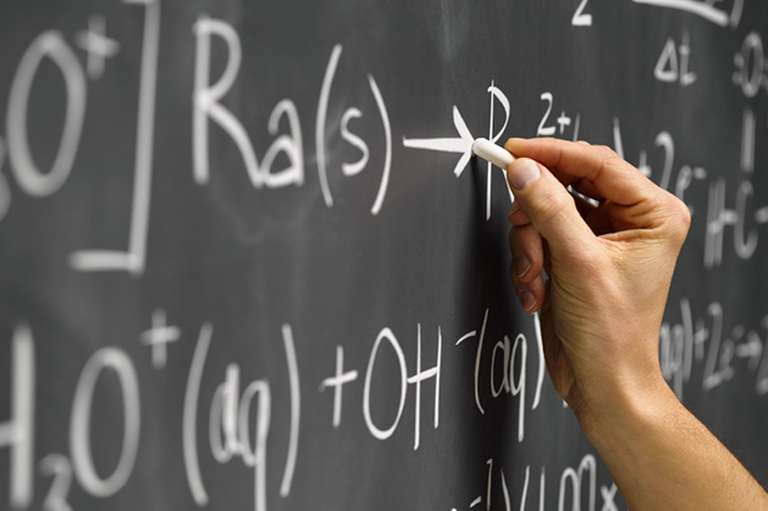# *General Education Courses*

Resources to help with foundation classes like English composition, math, algebra, and introduction to research.

## Mathematics: Description and Student Learning OutcomesMathematics is the study of numerical relationships, geometrical and spatial properties, quantitative reasoning, and logic.  In the area of Mathematics, students will demonstrate basic math skills and apply quantitative reasoning.  Courses in this category provide students with learning experiences that include practical application of mathematical concepts.

Apply basic quantitative and critical reasoning to solve real-world problems.

Apply advanced quantitative and critical reasoning to solve real-world problems.

## Mathematics and Algebra

Welcome to the Mathematics pages!

Click on the tabs above to access helpful resources to assist you with your math assignments. Access additional pages devoted to Algebra and Statistics resources using the tabs on the left.

Need a Math Refresher?

Take a self-paced assessment in Learning Express Library to pinpoint where you may need refreshing.  Find tutorials and self-assessments in the Adult Core Skills > Build Your Math Skills module.Find ebooks on mathematics, algebra, and statistics by searching the following library databases.

Selections from the Online LibraryFind articles and other resources by searching the following library databases.  Consider using search terms such as:

• Math
• Mathematics
• Algebra
• Statistics• View this FAQ for step-by-step instructions for setting up a Symbolab account.
• Unsure of whether or not a website you found is credible and fit to use for an assignment? Visit this Answer!Need help with math? Looking to brush-up on your math knowledge? Looking for a way to make math fun?Learning ExpressMath WayMicrosoft Math SolverPhoto Math